# edge-coloring

## Edge-antipodal colorings of cubes ★★

Author(s): Norine

We letdenote the-dimensional cube graph. A mapis called edge-antipodal if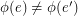wheneverare antipodal edges.

Conjecture   If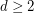andis edge-antipodal, then there exist a pair of antipodal vertices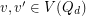which are joined by a monochromatic path.

Keywords: antipodal; cube; edge-coloring

## Goldberg's conjecture ★★★

Author(s): Goldberg

The overfull parameter is defined as follows: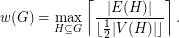Conjecture   Every graphsatisfies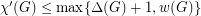.

Keywords: edge-coloring; multigraph

## Seymour's r-graph conjecture ★★★

Author(s): Seymour

An-graph is an-regular graphwith the property that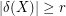for everywith odd size.

Conjecturefor every-graph.

Keywords: edge-coloring; r-graph

## Monochromatic reachability or rainbow triangles ★★★

Author(s): Sands; Sauer; Woodrow

In an edge-colored digraph, we say that a subgraph is rainbow if all its edges have distinct colors, and monochromatic if all its edges have the same color.

Problem   Letbe a tournament with edges colored from a set of three colors. Is it true thatmust have either a rainbow directed cycle of length three or a vertexso that every other vertex can be reached fromby a monochromatic (directed) path?

Keywords: digraph; edge-coloring; tournament

## Monochromatic reachability in edge-colored tournaments ★★★

Author(s): Erdos

Problem   For every, is there a (least) positive integerso that whenever a tournament has its edges colored withcolors, there exists a setof at mostvertices so that every vertex has a monochromatic path to some point in?

Keywords: digraph; edge-coloring; tournament

## Weak pentagon problem ★★

Author(s): Samal

Conjecture   Ifis a cubic graph not containing a triangle, then it is possible to color the edges ofby five colors, so that the complement of every color class is a bipartite graph.

## List colorings of edge-critical graphs ★★

Author(s): Mohar

Conjecture   Suppose thatis a-edge-critical graph. Suppose that for each edgeof, there is a listofcolors. Thenis-edge-colorable unless all lists are equal to each other.

Keywords: edge-coloring; list coloring

## A generalization of Vizing's Theorem? ★★

Author(s): Rosenfeld

Conjecture   Letbe a simple-uniform hypergraph, and assume that every set of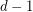points is contained in at mostedges. Then there exists an-edge-coloring so that any two edges which sharevertices have distinct colors.

Keywords: edge-coloring; hypergraph; Vizing

## Partitioning edge-connectivity ★★

Author(s): DeVos

Question   Letbe an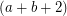-edge-connected graph. Does there exist a partitionof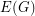so thatis-edge-connected andis-edge-connected?

Keywords: edge-coloring; edge-connectivity

## Acyclic edge-colouring ★★

Author(s): Fiamcik

Conjecture   Every simple graph with maximum degreehas a proper-edge-colouring so that every cycle contains edges of at least three distinct colours.

Keywords: edge-coloring